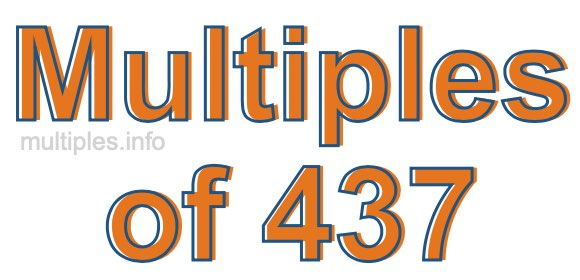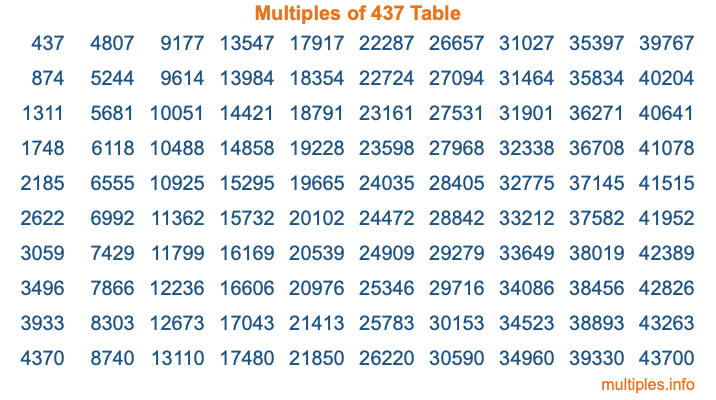Multiples of 437Welcome to the Multiples of 437 page. Here we will first teach you everything you will ever need to know about the multiples of 437, and then give you a study guide summary of everything we taught you to make sure you remember it all. Use this page to look up facts and learn information about the multiples of 437. This page will make you a multiples of four hundred thirty-seven expert!

Definition of Multiples of 437
Multiples of 437 are all the numbers that when divided by 437 equal an integer. Each of the multiples of 437 are called a multiple. A multiple of 437 is created by multiplying 437 by an integer.

Therefore, to create a list of multiples of 437, you start with 1 multiplied by 437, then 2 multiplied by 437, then 3 multiplied by 437, and so on for as long as you want. Thus, the list of the first five multiples of 437 is 437, 874, 1311, 1748, and 2185. To see a larger list of multiples of 437, see the printable image of Multiples of 437 further down on this page. We also have a category where you can choose any nth multiple of 437.

Multiples of 437 Checker
The Multiples of 437 Checker below checks to see if any number of your choice is a multiple of 437. In other words, it checks to see if there is any number (integer) that when multiplied by 437 will equal your number. To do that, we divide your number by 437. If the the quotient is an integer, then your number is a multiple of 437.

Is  a multiple of 437?

Least Common Multiple of 437 and ...
A Least Common Multiple (LCM) is the lowest multiple that two or more numbers have in common. This is also called the smallest common multiple or lowest common multiple and is useful to know when you are adding our subtracting fractions. Enter one or more numbers below (437 is already entered) to find the LCM.

Check out our LCM Calculator if you need more details about the Least Common Multiple or if you need the LCM for different numbers for adding and subtraction fractions.

nth Multiple of 437
As we stated above, 437 is the first multiple of 437, 874 is the second multiple of 437, 1311 is the third multiple of 437, and so on. Enter a number below to find the nth multiple of 437.

th multiple of 437

Multiples of 437 vs Factors of 437
437 is a multiple of 437 and a factor of 437, but that is where the similarities end. All postive multiples of 437 are 437 or greater than 437. All positive factors of 437 are 437 or less than 437.

Below is the beginning list of multiples of 437 and the factors of 437 so you can compare:

Multiples of 437: 437, 874, 1311, 1748, 2185, etc.

Factors of 437: 1, 19, 23, 437

As you can see, the multiples of 437 are all the numbers that you can divide by 437 to get a whole number. The factors of 437, on the other hand, are all the whole numbers that you can multiply by another whole number to get 437.

It's also interesting to note that if a number (x) is a factor of 437, then 437 will also be a multiple of that number (x).

Multiples of 437 vs Divisors of 437
The divisors of 437 are all the integers that 437 can be divided by evenly. Below is a list of the divisors of 437.

Divisors of 437: 1, 19, 23, 437

The interesting thing to note here is that if you take any multiple of 437 and divide it by a divisor of 437, you will see that the quotient is an integer.

Multiples of 437 Table
Below is an image of the first 100 multiples of 437 in a table. The table is in chronological order, column by column. The first column has the first ten multiples of 437, the second column has the next ten multiples of 437, and so on.The Multiples of 437 Table is also referred to as the 437 Times Table or Times Table of 437. You are welcome to print out our table for your studies.

Negative Multiples of 437
Although not often discussed or needed in math, it is worth mentioning that you can make a list of negative multiples of 437 by multiplying 437 by -1, then by -2, then by -3, and so on, to get the following list of negative multiples of 437:

-437, -874, -1311, -1748, -2185, etc.

Multiples of 437 Summary
Below is a summary of important Multiples of 437 facts that we have discussed on this page. To retain the knowledge on this page, we recommend that you read through the summary and explain to yourself or a study partner why they hold true.

There are an infinite number of multiples of 437.

A multiple of 437 divided by 437 will equal a whole number.

437 divided by a factor of 437 equals a divisor of 437.

The nth multiple of 437 is n times 437.

The largest factor of 437 is equal to the first positive multiple of 437.

437 is a multiple of every factor of 437.

437 is a multiple of 437.

A multiple of 437 divided by a divisor of 437 equals an integer.

437 divided by a divisor of 437 equals a factor of 437.

Any integer times 437 will equal a multiple of 437.

Multiples of a Number
Here you can get the multiples of another number, all with the same attention to detail as we did for multiples of 437 on this page.

Multiples of
Multiples of 438
Did you find our page about multiples of four hundred thirty-seven educational? Do you want more knowledge? Check out the multiples of the next number on our list!